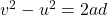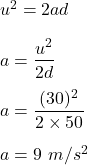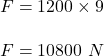## A car with a mass of 1,200 kg traveling westward at 30 m/s is slowed to a stop in a distance of 50 m by the car’s brakes. What w

Question

A car with a mass of 1,200 kg traveling westward at 30 m/s is
slowed to a stop in a distance of 50 m by the car’s brakes.
What was the braking force?

A. 10,800 N
B. 36.000 N
C. 6000 N
D. 9000 N

in progress 0
6 months 2021-07-24T19:11:40+00:00 1 Answers 175 views 0

## Answers ( )

F = 10800 N

Explanation:

We have,

Mass of a car, m = 1200 kg

Initial speed of the car, u = 30 m/s

Finally speed, v = 0 (as it stops)

Stopping distance, d = 50 m

It is required to find the braking force. For this firstly we can find the acceleration of the car using equation of kinematics as :Braking force is, F = maSo, the braking force is 10800 N. The correct option is A